## Validity Proven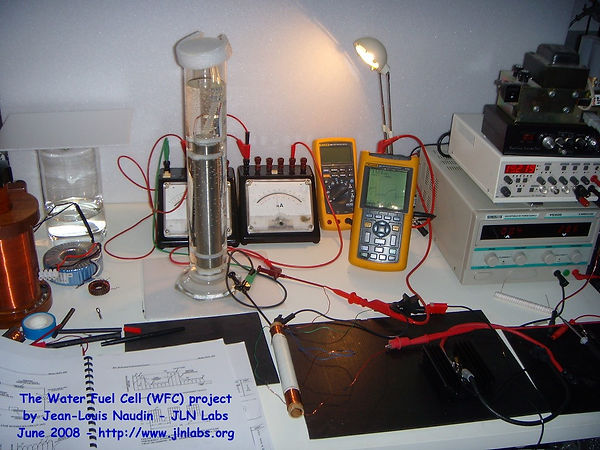There are thousands of builders now globally,

We invite you to invest and join in to also support the rise of this technology.

We show h2 some examples of the work carried out proving the technology rebuilding it and now mass deployment.## Working As Described University Report

The WFC is a water capacitor and uses ORDINARY TAP WATER without any electrolyte...

Comments : Test of the  WFC powered by Voltrolysis is very encouraging, it shows that it is indeed possible to produce quickly a great amount of HHO with only ordinary tap water without any electrolyte... Stanley A Meyer Told the Truth FACT

Links to Univesity Lab Testing , Replications and references:January 25, 2013 - TESTS02E01 :

Following the TEST  about the production of HHO gas,

I have continued the tests with the Stanley Meyer's Water Fuel Cell (WFC).

The TEST has demonstrated that High Voltage Nano Pulses at High Frequency produced   accross an electrolysis cell are able to produce very quickly a great amount of HHO gas.

According to these encouraging tests, I have reconducted my experiments done about the Stanley Meyer's WFC from June 2008.

I have replaced the VIC (Voltage Intensifier Circuit), a replication of the   Stanley A  Meyer's circuit,  with 100% positive results.

Dan

"I encourage all schools and builders to replicate and teach such technologies aggressively."Notes :

The use of pure osmosed water without electrolyte (not electrically conductible) is very important here, this has allowed to remove completly the HHO generation by electrolysis.

AS ZERO  SALTS ZERO ELECTROLYTES ONLY PURE OSMOSED WATER.

The WFC is a water capacitor, in this test it consumes too much current due to its impedance Vs the pulsed current.

During long duration tests (>6min) the WFC begins to heat up and thus, this confirms this fact. The effect discovered by Stanley Meyer seems really present here, because the pure osmosed water is split.The High Voltage pulse sent by the induction  accross the WFC via the flat bifilar coil need to be optimized so as to reduce the current. I think that the WFC must be used as a capacitor in a resonant RLC type circuit like the Meyer's VIC original circuit..SEE  9XB  and    9XA Pages

THE PARAMETRIC POWER CONVERSION

Created on April 16th, 1997 - JLN Labs - Last update : January 10th, 2003
Thanks to Fred B. EppsGreg WatsonEpitaxy

All informations in this page are published free and are intended for private/educational purposes and not for commercial applications

As shown by Equation #1, electromotive force, and hence the transfer of electrical energy can be achieved in two ways:

(1) by flux coupling,

or

(2) by parametric coupling.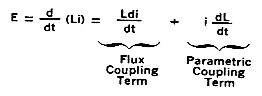##### Equation #1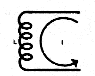Figure 1

Consider a simple inductance (L) carrying a current (i) as shown in fig 1.

One knows that there is energy stored in this inductance of magnitude 1/2 LI*2 and if this inductance is now increased in some manner, such as by inserting an iron core into L (while holding I constant). then the stored electrical energy is increased. This is an example of parametric power transfer. (see Equation 1)."NO energy is required to insert the iron core into the inductor.

It is ATTRACTED into the core and the resultant increased coil energy is pumped back into the power source (coil current drops)!" (Greg Watson)

"No mechanical energy input is required to insert the iron core into the inductor, BECAUSE iron core is attracted by the inductor itself, thus no mechanical energy need to be expended !" (Epitaxy)

If in accordance whith Fig 2, the afore-mentionned core is now alternately inserted into and withdrawn from the inductance L and this inductance is, in turn a part of tank circuit, then a parametric oscillator result.Figure 2

The most critical relationship associated with this parametric oscillator relate to frequency. For such devices, it is a well known fact that ideally, the pumping frequency (rotating eccentric) should be twice the frequency to which the resonant tank is tuned ( fig 3 ).Fig 3

The following example demonstrate the general validity of this statement :

Assume that the current in the tank is oscillating at one-half the frequency of rotating eccentric ( see fig 4 ).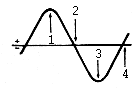Fig 4

Current in LC TankReferring to fig 4, if the core is inserted at point (1) in the cycle, this increase in L, coupled with the large current flowing at that time, results in a large parametric energy transfer from the mechanism to the circuit.

Furthermore, if the core is now withdrawn one-half of a mechanical cycle later (or one-quarter of an electrical cycle at point (2), the current is now zero and no electrical energy is withdraw from the tank. Similarly at point (3) we have additional parametric energy transfer and at (4) no transfer.

Thus, by doubling the pumping frequency, power can be unilaterally transferred parametrically in accordance with the second term of Equation 1.Fig 5
Parametric amplification of energyFig 6
Transient state

( start of parametric amplification )

In the example cited above, a mechanical driver was used. The final step, of course, is to achieve a simple passive all-electrical parametric device in which the double frequency pumping action is an intrinsic part of the device.

( Ref document : The PARAFORMER (TM), "A new passive power conversion device" by Dr S.D.Wanlass and Dr L.K. Wanlass )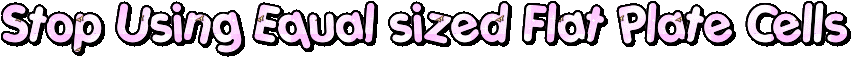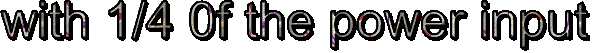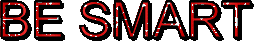##### "proof of concept" experiment.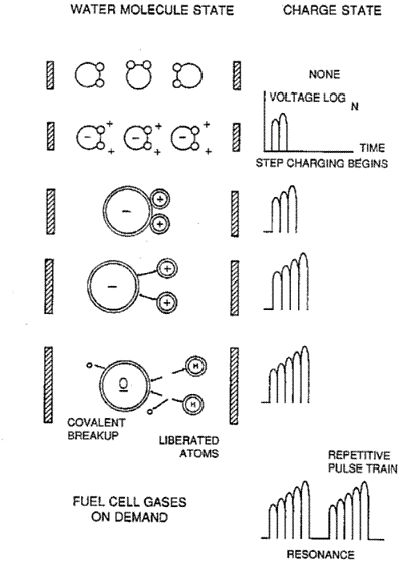Preliminary tests of the 1st WFC v1.0 ( June 12, 2008):

Below, the video of the experimental WFC v1.0 working in resonance mode with distilled water. A high flow of gases is produced at the WFC v1.0 resonance frequency.

You may notice that, in this case, the size of the bubbles produced are very tiny compared to a common electrolysis process.

Here is the Water Fuel Cell, the most important thing is that the WFC uses only ORDINARY TAP WATER without need of ELECTROLYTE for working properly. The stainless steel electrodes are the main parts of a water capacitor.###### The High Frequency is rectified via an ultra fast HFA25PB60 diode so as to produce High Frequency DC pulses accross the WFC.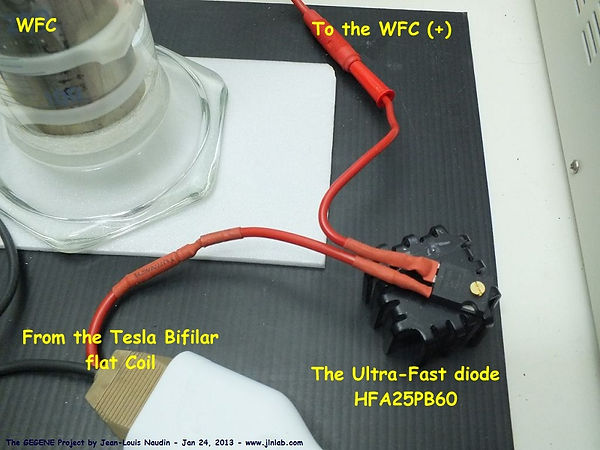The full datasheet of the diode can be found HERE

Below the details of the WFC cell used for this "proof of concept" experiment.# Parametric Resonance

This demonstration illustrates parametric resonance, which is a resonance phenomenon that arises because some parameter of the system is varying periodically in time.

This happens, for example, when you periodically extend and retract your legs at an appropriate frequency while sitting on a playground swing. If a pendulum consists of a mass connected to a frictionless hinge by an idealized rod of length L, then the angle θ between the rod and vertical obeys the differential equation

θ" + (g/L) sinθ=0

If the length of the pendulum varies with time, the differential equation becomes

θ" + 2(L'(t)/L(t))θ'+(g/L(t)) sinθ=0

In this Applet, we choose the length of the pendulum to be L(t)=1+A cos(wt) and we choose g=4π2. With this choice of g, solutions of the A=0, linearized equation θ"+gθ=0 have period 1.

At time t, the length of the blue line in the Applet below is L(t). The angle between the blue line and vertical is θ(t). For comparison purposes, the gray pendulum provides the same data for the A=0, g=4π2, linearized equation

θ" + g θ =0

So, at time t, the length of the gray line is 1 and the angle between the gray line and vertical is a constant (determined by the initial speed that I have chosen) times sin(2πt).

The demonstration below is an applet. Google Chrome, Firefox and Microsoft Edge no longer execute applets because of security issues with NPAPI plugins. Some browsers that still play applets are Internet Explorer,

Safari and the Pale Moon browser [ windows, linuxMac ].

easy.Parametric resonance tests of the WFC at 2*fo and at 0.5*fo :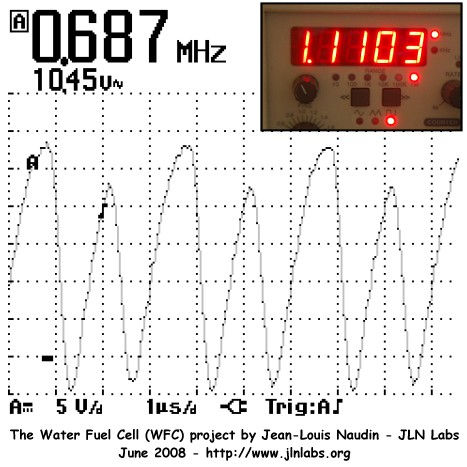When the frequency of the pump is set to Fpump = 2*fo = 1.110 MHz
the resonance of the WFC is fo= 0.687 MhzWhen the frequency of the pump is set to Fpump = 0.5*fo = 0.329 MHz
the resonance of the WFC is fo= 0.560 Mhz

#### Water Fuel Technology Works### DBD BarrierThe center electrode of the WFC v1.0 has been covered with a thin adhesive plastic sheet so has to get a fully insulated cathod.

Thank You Hydrogen Hot Rodders.

So, it is now possible to get the Voltage Intensification with an electrical step charging effect.

Below the measured specs of the new insulated WFC v1.1.

## ​Above

: The WFC 1.1 has been tested with 30V

squared pulses at 105 Hz.
When the voltage is switched off, the WFC acting as a water capacitor, discharges on itself.